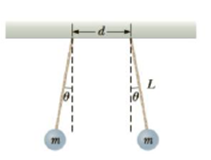Chapter 15, Problem 65AP

Chapter
Section
Textbook Problem

Two hard rubber spheres, each of mass m = 15.0 g, are rubbed with fur on a dry day and are then suspended with two insulating strings of length L = 5.00 cm whose support points axe a distance d = 5.00 cm from each other as shown in Figure P15.65. During the rubbing process, one sphere receives exactly twice the charge of the other. They are observed to hang at equilibrium, each at an angle of θ = 10.0° with the vertical. Find the amount of charge on each sphere.Figure P15.65

To determine
The charge on each sphere.

Explanation

Given info: The mass of the sphere is 15.0 g. The length of the string is 5.00 cm. The magnitude of one charge is twice the other.

The free body diagram is,

• FE is the net electric force.
• T is the tension.
• m is the mass of the ball.
• g is the acceleration due to gravity.

From the diagram,

Tcos10ο=mg

Tsin10ο=FE

Dividing the 2nd equation by the 1st,

FE=mgtan10ο

Formula to calculate the net electric force is,

FE=ke(q)(2q)r2

• ke is the Coulomb constant.
• r is the distance between the spheres.
• q is the magnitude of smaller charge.

The distance between the spheres is,

r=d+2Lsin10ο

Therefore,

2keq2(d+2Lsin10ο)2=mgtan10ο

On Re-arranging,

q=(d+2Lsin10ο)mgtan10ο2ke

Substitute 9.8ms2 for g, 15

Still sussing out bartleby?

Check out a sample textbook solution.

See a sample solution

The Solution to Your Study Problems

Bartleby provides explanations to thousands of textbook problems written by our experts, many with advanced degrees!

Get Started